Request a call back

# Most Important Questions for CBSE Class 10 Mathematics 2021

Chapter 1: Real Numbers

1. By what number should 1365 be divided to get 31 as the quotient and 32 as the remainder?                                 

2. If HCF (26, 169) = 13, then find LCM (26, 169).                                                                                                 

3. Giventhat  is irrational, prove thatis an irrational number.                                                            

4. Without actually performing long division, state whether the following rational numbers will have a terminating decimal expansion or a non-terminating repeating decimal expansion:5. Find the largest number which divides 378 and 510 leaving the remainder 6 in each case.                                      

6. Three sets of English, Mathematics and Science books containing 336, 240 and 96 books, respectively, have to be stacked in such a way that all the books are stored subject wise and the height of each stack is the same. How many stacks will be there?                                                                                                                                                                              

7. Prove that is an irrational number.                                                                                                                     

Chapter 2: Polynomials

1.Divide the polynomial p(x) by the polynomial g(x) and find the quotient and remainder.                                                                                                                                                         

2. Ifand –3 are the zeroes of the polynomial ax2 + 7x + b, then find the values of a and b.                                 

3. Obtain all other zeroes of, if two of its zeroes are.                           

4. If the polynomialis divided by another polynomial , the remainder comes out to be x + a. Find k and a.                                                                                                                                                                 

Chapter 3: Pair of Linear Equations in Two Variables

1. A fraction becomesif 2 is added to both its numerator and denominator. If 3 is added to both its numerator and denominator, then it becomes. Find the fraction.                                                                                              

2. Solve  the following system of equations graphically:

2x + 3y = 2

x − 2y = 8                                                                                                                                                      

3. 2 men and 5 boys complete a piece of work in 4 days, while 3 men and 6 boys complete it in 3 days. Find the time taken by one man alone and one boy alone to complete the work.                                                                                        

4. A boat goes 12 km upstream and 40 km downstream in 8 hours. It can go 16 km upstream and 32 downstream in the same time. Find the speed of the boat in still water and the speed of the stream.                                                              

1. Find the discriminant of the following equation: 3x2 –2x + 8 = 0                                                                        

2. Find the value of k for which x = 1 is a root of the equation x2 + kx + 3 = 0                                                       

3. Using completing the square method, show that the equation x2 – 8x + 18 = 0 has no solution.                           

4. Solve the following quadratic equation:5. Solve the quadratic equation by the formula method. x+ 3x – 7 = 0                                                                 

6. Show that the following equation has real roots, and solve by using the quadratic formula:  3x2 – 32x + 12 = 0     

7. Solve the following quadratic equation:8. A sailor can row a boat 8 km downstream and return to the start point in 1 hour 40 minutes. If the speed of the stream is 2 kmph, then find the speed of the boat in still water.                                                                                               

Chapter 5: Arithmetic Progression

1. Find the 6th term from the end of the AP 5, 7, 9,….201.                                                                                      

2. The sum of three numbers in AP is 3 and their product is −35. Find the common difference.                                  

3. The 7th term of an AP is -4 and its 13th term is -16. Find the AP.                                                                         

4. If the pth term of an AP is q and its qth term is p, then show that its (p + q)th term is zero.                                   

5. The 4th term of an AP is zero. Prove that its 25th term is triple its 11th term.                                                        

6. How many terms of the AP : 24, 21, 18,… must be taken so that their sum is 78?                                                 

7. The sum of three terms of an A.P. is 21 and the product of the first and the third terms exceeds the second term by 6, find three terms.                                                                                                                                                        

8. The sum of n terms of two A.P.’s are in the ratio 3n + 5:5n + 7. Then, find the ratio of their nth term.                    

Chapter 6: Triangles

1. Given ∆ABC ∼ ∆PQR,if  then find2. In the given figure, ∆OAB ~∆OCD. If AB = 8 cm, BO = 6.4 cm, OC = 3.5 cm and CD = 5 cm,

find (i) OA and (ii) DO.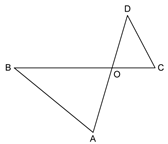3. In the given figure, O is a point inside ∆PQR such that ∠ POR = 90°, OP = 6 cm and OR = 8 cm. If PQ = 24 cm and QR = 26 cm, prove that ∆PQR is right angled.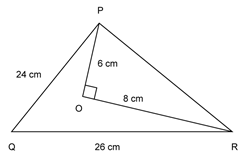4. If a line is drawn parallel to one side of a triangle to intersect the other two sides in distinct points, prove that the other two sides are divided in the same ratio.                                                                                                                          

5. Prove that in a right triangle, the square of the hypotenuse is equal to the sum of the squares of the other two sides. 

6. AD is a median of a triangle ABC and AM is perpendicular to BC. Prove that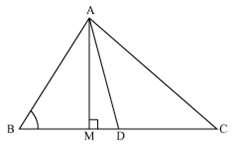1.2.3.Chapter 7: Coordinate Geometry

1. The line segment joining the points (3, –4) and (1, 2) is trisected at points P(a, -2) and Qthen find the values of a and b.                                                                                                                                                                         

2. If A(6, -1), B(1, 3) and C(k, 8) are three points such that AB = BC, find the value of k.                                           

3. Given figure shows the arrangement of desks in a classroom. Anita, Atharva and Atish are seated at A, B and C respectively. Do you think they are seated in a line? Give reasons for your answer.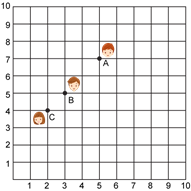4. Show that the points A(2, 1), B(5, 2), C(6, 4) and D(3, 3) are the angular points of a parallelogram. Is this figure a rectangle?                                                                                                                                                                             

5. Find the ratio in which the point P(m, 6) divides the line joining A(–4, 3) and B(2, 8). Also, find the value of m.       

Chapter 8: Introduction to Trigonometry

1. Show that.                                                                          

2. If ∆ABC is right angled at C, then find the value of cos (A + B).                                                                            

3. If cosec= 3x and cot, then find the value of4. Prove that5. If tan, show that6. If x = cot A +cos A and y =cot A-cos A, then show thatChapter 9: Some Applications of Trigonometry

1. A tower stands vertically on the ground. From a point on the ground which is 20 m away from the foot of the tower, the angle of elevation of its top is found to be 60°. Find the height of the tower.2. As observed from the top of a lighthouse, 100 m above sea level, the angle of depression of a ship sailing directly towards the lighthouse changes from 30° to 60°. Determine the distance travelled by the ship during the period of observation.3. Two windmills of height 50 m and 40 m are on either side of the field. A person observes the top of the windmills from a point in between the towers. The angle of elevation was found to be 45° in both the cases. Find the distance between the windmills.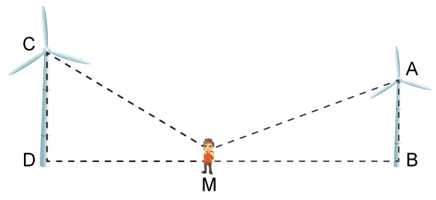4. The angle of elevation of a jet plane from a point A on the ground is 60°. After a flight of 15 seconds, the angle of elevation changes to 30°. If the jet plane is flying at a constant height of , find the speed of the jet plane is flying at a constant height offind the speed of the jet plane.                                                                                                           

5. The angle of elevation of the top of a building from a point A on the ground is 30°. On moving a distance of 30 m towards its base to a point B, the angle of elevation changes to 45°. Find the height of the building and the distance of its base from the point A.Chapter 10: Circles

1. Prove that the lengths of the tangents drawn from an external point to a circle are equal.                                      

2. Prove that the tangents drawn at the end points of a chord of a circle make equal angles with the chord.                

3. Out of the two concentric circles, the radius of the outer circle is 5 cm and the chord AC of length 8 cm is a tangent to the inner circle. Find the radius of the inner circle.                                                                                                                

4. In Fig.3, from an external point P, two tangents PT and PS are drawn to a circle with centre O and radius r. If OP = 2r, show that ∠ OTS = ∠ OST = 30°.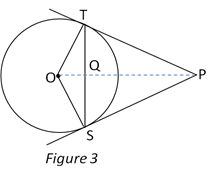Chapter 11: Constructions

1. Draw a line segment of length 7.6 cm and divide it in the ratio 5:8. Measure the two parts.                                     

2. Draw a circle of radius 4.2 cm. Draw a pair of tangents to this circle inclined to each other at an angle of 45°.          

Chapter 12: Areas Related to Circles

1. The areas of two concentric circles are 962.5 cm2 and 1386 cm2. Find the width of the ring.                                   

2. In the given figure, AB is a diameter of a circle with centre O and OA = 7 cm. Find the area of the shaded region.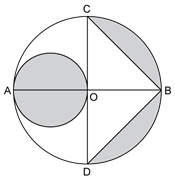3. Find the area of the shaded region shown in the given figure. The four corners are circle quadrants, and at the centre, there is a circle. (Take p = 3.14)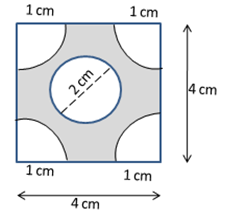4. A lawn is rectangular in the middle, and it has semicircular portions along the shorter sides of the rectangle. The rectangular portion measures 50 m by 35 m. Find the area of the lawn.                                                                                     

Chapter 13: Surface Areas and Volumes

1. A bucket made of a metal sheet is in the form of a frustum of a cone of height 16 cm with radii of its lower and upper ends as 8 cm and 20 cm, respectively. Find the cost of the bucket if the cost of the metal sheet used is Rs. 15 per 100 cm2. Use.                                                                                                                                                                              

2. A solid metal cone with radius of base 5 cm and height 12 cm is melted to form solid spherical balls of diameter 4 cm each. The number of balls will be formed?                                                                                                                               

3. A solid is composed of a cylinder with hemispherical ends. If the whole length of the solid is 98 cm and the diameter of each of its hemispherical ends is 28 cm, find the cost of polishing the surface of the solid at the rate of 15 paise per sq. cm. Use.                                                                                                                                                                               

4. A farmer connects a pipe of internal diameter 25 cm from a canal to a cylindrical tank in his field which is 12 m in diameter and 2.5 m deep. If water flows through the pipe at the rate of 3.6 km/h, in how much time will the tank be filled? Also, find the cost of water if the water supply department charges at the rate of Rs. 0.07. Use.                                                   

5. A wooden article was made by scooping out a hemisphere from each end of a solid cylinder, as shown in the figure. If the height of the cylinder is 12 cm and its base is of radius 4.2 cm, find the total surface area of the wooden article. Also, find the volume of the wood left in the article. Use.                                                                                                 

Chapter 14: Statistics

1. Find the mean using the direct method:                                                                                                               

 Class Frequency 10–20 20–30 30–40 40–50 50–60 60–70 11 15 20 30 14 10

2. Find the missing frequenciesin the table given below. It is given that the mean of the given frequency distribution is 50.                                                                                                                                                                       

 Class Frequency 0–20 20–40 40–60 60–80 80–100 1732190 Total 120

3. Calculate the median from the following frequency distribution:                                                                             

 Class Frequency 5–10 10–15 15–20 20–25 25–30 30–35 35–40 40–45 5 6 15 10 5 4 2 2

Chapter 15: Probability

1. 250 lottery tickets were sold and there are 5 prizes on these tickets. If Kunal has purchased one lottery ticket, what is the probability that he wins a prize?                                                                                                                              

2. A game consists of tossing a one rupee coin 3 times and nothing its outcome each time. Harsh wins if all the tosses give the same result. i.e. three heads or three tails, and loses otherwise. Calculate the probability that Harsh will lose the game. 

3. A card is drawn at random from a well-shuffled deck of playing cards. Find the probability of drawing (i) a face card, (ii) a card which is neither a king nor a red card.                                                                                                                       

4. Two dice are thrown simultaneously. What is the probability that

1. 5 will not come up on either of them
2. 5 will not come up on at least one
3. 5 will come up at both dice                                                                                                                            

5. Tickets numbered 2, 3, 4, 5, …, 100, 101 are placed in a box and mixed thoroughly. One ticket is drawn at random from the box. Find the probability that the number on the ticket is

1. An even number
2. A number less than 16
3. A number which is a perfect square
4. A prime number less than 40                                                                                                                        

Case Study

Case study based questions are compulsory and of 4 marks. Attempt any four sub parts of each question. Each subpart carries 1 mark.

1. Case Study based – 1

Similar Triangles and their Properties

After having a heavy discussion, the Members of the community has decided to build a community garden in Shikha’s neighborhood. The garden is triangular in shape and it is surrounded by a fence. Few hours later, they decides to build one more garden of the same shape with different measurements besides the previous one. The overall structure and measurements of the gardens are shown in the picture.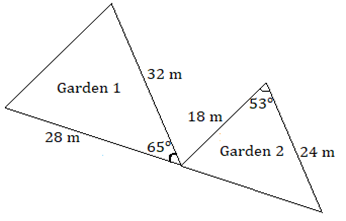a) What will be the measurements of the two unknown angles of the larger garden?

1. 53° and 62°
2. 65° and 53°
3. 62° and 65°
4. 60° and 55°

b) The measurements of the other two angles of the smaller garden are

1. 53° and 62°
2. 65° and 53°
3. 62° and 65°
4. 60° and 55°

c) Ratio of the area of larger garden to that of the area of smaller garden is

1. 16 : 9
2. 9 : 16
3. 4 : 3
4. 3 : 4

d) Find the unknown side of the larger garden.

1. 12m
2. 24m
3. 36m
4. 48m

e) If the unknown side of garden 2 is 21 m then find the Area covered by the smaller garden.

1.2.3.4.2. Case Study based – 2

Cubic Polynomials

Rohan saw that an electric wire got bent due to heavy storm as shown in the figure. He claimed that it looks like a mathematical shape. Answer the following questions below asked by Rohan.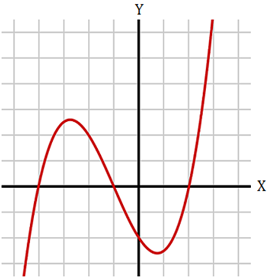Scale, 1 cm = 1 unit

a) The number of zeroes possible for the polynomial made by the wire is

i. 1         ii. 2          iii. 3           iv. 4

b) Zeroes of the polynomial are

i. –4, 1 and 2     ii. –2 and 0     iii. –3, 1 and 2    iv. –4, –1 and 2

c) What will be the degree of the polynomial?

i. 0         ii. 1        iii. 2         iv. 3

d) Which of the following can be a polynomial (made by the wire) represented in the graph?

i. x3 + 5x2 + 2x – 8                     ii.    x3 + 3x2 – 6x – 8

iii.   x3 + 3x2 + 2x – 9                 iv.   x3 + x2 – 6x – 8

e) Value of the polynomial at the point where x will be –2 is

i. 0           ii.  –2           iii.  2            iv.  –4

3. Case Study Based – 3

Relay Race

On sports day, a relay race of 400 m has been conducted by the School. To measure the exact time took by each group of students, a stopwatch was used and the timing were recorded in tabular form as shown: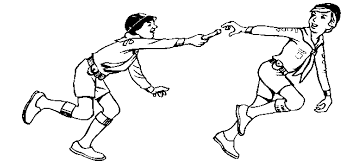Time(in sec) 120 – 140 140 – 160 160 – 180 180 – 200 200 – 220 No. of groups 2 5 4 3 1

a) Estimate the median time taken by a group to finish the race.

1. 167.5 seconds
2. 155.7 seconds
3. 234.5 seconds
4. 176.5 seconds

b) What will be the upper limit of the modal class?

1. 140
2. 160
3. 180
4. 200

c) Which of the following are measures of Central Tendency?

1. Mean
2. Median
3. Mode
4. All of the above

d) The sum of lower limits of median class and modal class is

1. 100
2. 200
3. 300
4. 400

e) How many groups finished the race within 3 minutes?

1. 2
2. 5
3. 7
4. 11

4. Case Study Based – 4

Applications of Surface Area and Volume

A school conduct educational trip every year for the students of class 10. Mathematics teacher took all her students to show Taj Mahal. As she had interest in History as well, she narrated the facts of Taj Mahal to students including that it is a combination of solid figures. Four cylindrical pillars (2 at the back and 2 at the front) are involved in the design along with two hemispherical domes at the corners. Also, there is one big dome at the centre.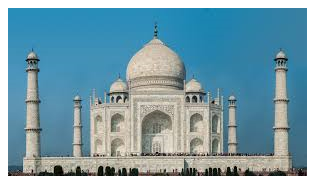a) How much fencing material will be required to cover the 2 small domes each with radius 3.5 m?

1. 154 m2
2. 245 m2
3. 345 m2
4. 235 m2

b) What will be the total surface area of the two cylindrical pillars (at the back) with height 14 m and radius 5 m each?

1. 1294.28 m2
2. 1194.28 m2
3. 597.14 m2
4. 1193.28 m2

c) Find the lateral surface area of the two cylindrical pillars with height 14 m and radius 5 m each?

1.  220m2
2.  440m2
3.  660m2
4.  880m2

d) Volume of one dome when the radius is 4.2 m will be

1. 115.232 m3
2. 165.242 m3
3. 155.232 m3
4. 195.232 m3

e) Find the volume of the 2 pillars (at the front) if each has height 16 m and radius 7m.

1. 1408 m3
2. 2816 m3
3. 4928 m3
4. 5816 m3

5. Case Study based – 5

SUN ROOM

The diagrams show the plans for a sun room. It will be built onto the wall of a house. The four walls of the sunroom are square clear glass panels. The roof is made using

• Four clear glass panels, trapezium in shape, all the same size
• One tinted glass panel, half a regular octagon in shape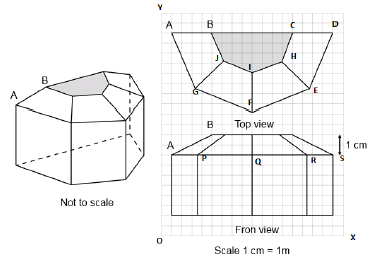(a) Refer to Front View

Find the mid-point of the segment joining the points J (6, 17) and I (9, 16).

(i)    (33/2, 15/2)

(ii)   (3/2, 1/2)

(iii)  (15/2, 33/2)

(iv)  (1/2, 3/2)

(b) Refer to Front View

The distance of the point P from the y-axis is

(i) 4 (ii) 15 (iii) 19 (iv) 25

(c) Refer to Front View

The distance between the points A and S is

(i) 4 (ii) 8 (iii)16 (iv)20

(d) Refer to Front View

Find the co-ordinates of the point which divides the line segment joining the points A and B in the ratio 1:3 internally.

(i) (8.5, 2.0) (ii) (2.0, 9.5) (iii) (3.0, 7.5) (iv) (2.0, 8.5)

(e) Refer to Front View

If a point (x, y) is equidistant from the Q(9, 8) and S(17, 8), then

(i) x + y = 13 (ii) x – 13 = 0 (iii) y – 13 = 0 (iv) x – y = 13

6. Case Study Based- 6

SCALE FACTOR AND SIMILARITY

SCALE FACTOR

A scale drawing of an object is the same shape as the object but a different size.

The scale of a drawing is a comparison of the length used on a drawing to the length it represents. The scale is written as a ratio.

SIMILAR FIGURES

The ratio of two corresponding sides in similar figures is called the scale factor.
Scale factor =If one shape can become another using Resizing then the shapes are Similar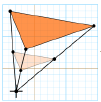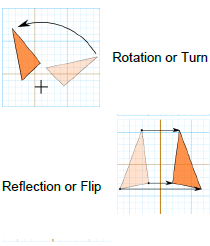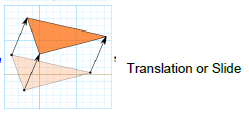Hence, two shapes are Similar when one can become the other after a resize, flip, slide or turn.

(a) A model of a boat is made on the scale of 1:4. The model is 120cm long. The full size of    the boat has a width of 60cm. What is the width of the scale model?

1. 20cm
2. 25cm
3. 15cm
4. 240cm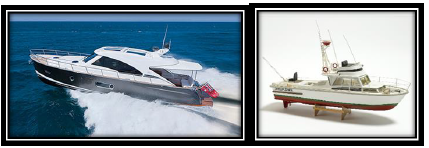(b)   What will affect the similarity of any two polygons?

1.   They are flipped horizontally
2.   They are dilated by a scale factor
3.   They are translated down
4.   They are not the mirror image of one another

(c)   If two similar triangles have a scale factor of a: b. Which statement regarding the two triangles is true?

1.  The ratio of their perimeters is 3a: b
2.  Their altitudes have a ratio a: b
3.  Their medians have a ratio 𝑎/2: b
4.  Their angle bisectors have a ratio a2: b2𝑎

(d)   The shadow of a stick 5m long is 2m. At the same time the shadow of a tree 12.5m high is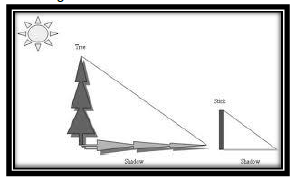1. 3m
2. 3.5m
3. 4.5m
4. 5m

(e) Below you see a student's mathematical model of a farmhouse roof with measurements. The attic floor, ABCD in the model, is a square. The beams that support the roof are the edges of a rectangular prism, EFGHKLMN. E is the middle of AT, F is the middle of BT, G is the middle of CT, and H is the middle of DT. All the edges of the pyramid in the model have length of 12 m.